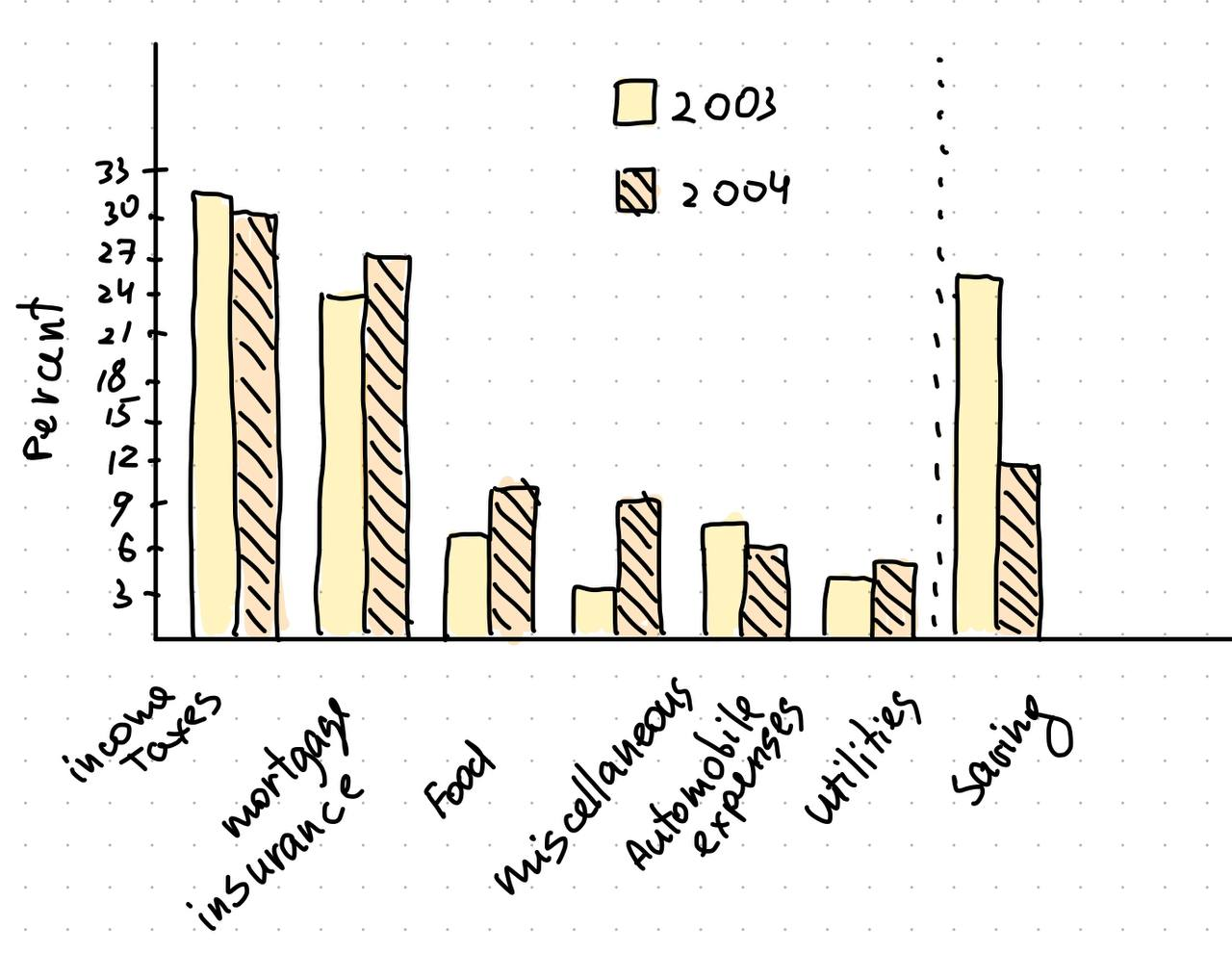# Get help with bar graphs problems

Recent questions in Bar GraphsMayra Berry 2022-06-10 Answered

### Prove that if diam(G)=3 for a regular graph G, then $diam\left(\overline{G}\right)=2.$.I know that without using regularity one can show that if . How do I use regularity to show that it must be exactly 2?Layla Velazquez 2022-06-07 Answered

### Boxplots and bar graphsI'm studying for the GRE and came across several questions that I was unable to answer in a practice booklet, even after looking at the answer and trying to work backwards, and searching google and other sites for helpful hints. I think I am missing a fundamental understanding or useful heuristic for solving many of these problems; any advice would be greatly appreciated as my exam is Monday (Aug 1st).1. Eight hundred insects were weighed, and the resulting measurements, in milligrams, are summarized in the boxplot below.If the 80th percentile of the measurements is 130 milligrams, about how many measurements are between 126 milligrams and 130 milligrams?I calculated the range (41), the quartiles(Q1=114, Q2=118, Q3=126), and the IQR (12), but I'm confused about the question. If the 80th percentile (so 80% of the measurements?) is at 130, then 640 are within this percentile. I'm not sure if this is true and even if it is, where to go from here. Each quartile is 25% of the data, correct? So from 126 to 146 must contain 25%, or 200 measurements? (1-.8)(200) = 40, but conceptually I'm lacking what that means.2.This question refers to the following graph:(a) In 2003 the family used a total of 49 percent of its gross annual income for two of the categories listed. What was the total amount of the family’s income used for those same categories in 2004 ?My confusion lies with the fact that I can't seem to find any combination of two categories that add up to 49%. Plus, it seems that the total % expenditure in each year is 101%. The chart is not 100% accurately drawn, but even being very liberal in measuring there seems to be a discrepancy.agrejas0hxpx 2022-04-06 Answered